GMAT  >  Test: Rectangular Solids- 1

# Test: Rectangular Solids- 1

Test Description

## 15 Questions MCQ Test Quantitative for GMAT | Test: Rectangular Solids- 1

Test: Rectangular Solids- 1 for GMAT 2023 is part of Quantitative for GMAT preparation. The Test: Rectangular Solids- 1 questions and answers have been prepared according to the GMAT exam syllabus.The Test: Rectangular Solids- 1 MCQs are made for GMAT 2023 Exam. Find important definitions, questions, notes, meanings, examples, exercises, MCQs and online tests for Test: Rectangular Solids- 1 below.
Solutions of Test: Rectangular Solids- 1 questions in English are available as part of our Quantitative for GMAT for GMAT & Test: Rectangular Solids- 1 solutions in Hindi for Quantitative for GMAT course. Download more important topics, notes, lectures and mock test series for GMAT Exam by signing up for free. Attempt Test: Rectangular Solids- 1 | 15 questions in 30 minutes | Mock test for GMAT preparation | Free important questions MCQ to study Quantitative for GMAT for GMAT Exam | Download free PDF with solutions
 1 Crore+ students have signed up on EduRev. Have you?
Test: Rectangular Solids- 1 - Question 1

### A rectangular container X has a total capacity of 5 cubic feet. If each dimension of X is multiplied by a positive number D, then a new rectangular container Y is formed. If Y has a total capacity of 135 cubic feet. What is the value of D?

Test: Rectangular Solids- 1 - Question 2

### Area of 3 adjacent faces of a rectangular solid are x2, 4x and 16x respectively where ‘x’ is a positive integer. What is the volume of this solid (in terms of x)?

Test: Rectangular Solids- 1 - Question 3

### What is the largest possible diagonal (in cms) of a fish tank (in the form of a rectangular solid), if it is 20cms long, 10 cms wide and 20 cms high?

Test: Rectangular Solids- 1 - Question 4

1/3rd of a large can X in the shape of a right circular cylinder is filled with cola. If the volume of cola in the can is 12 cubic inches and the radius of the can is 6 inches, then what is the height of the can X?

Test: Rectangular Solids- 1 - Question 5

A large can in the shape of a right circular cylinder has a total capacity of 16π cubic inches. When completely filled, this cylinder can fill to capacity exactly 4 equal smaller cans (right circular cylinder) of height 1 inch each. What is the radius (in inches) of a smaller can?

Test: Rectangular Solids- 1 - Question 6

A certain right circular cylinder is filled halfway with g gallons of water. When placed on its side, the maximum depth of the water is c centimeters. In terms of c and g , what is the height of the cylinder?

Detailed Solution for Test: Rectangular Solids- 1 - Question 6

Step 1: Question statement and Inferences

A certain right circular cylinder is filled halfway with g gallons of water, so its volume is 2g. When placed on its side, the maximum depth of the water is c centimeters, so its radius is c.

Step 2: Finding required values

Set up the equation to solve for h. The volume of any right circular cylinder is V = πr2h. Knowing that V = 2g and that c = r, set up the equation.

Step 3: Calculating the final answer

Set up the equation like this:

2g = πc2h

To isolate h, divide both sides by πc2

h=2g/πc2

Test: Rectangular Solids- 1 - Question 7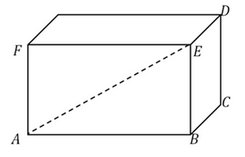In the rectangular solid above, BCDE is a square and the dotted line connecting A to E is twice the length of edge EB. If edge AB is √3, what is the volume of the solid?

Detailed Solution for Test: Rectangular Solids- 1 - Question 7

Step 1: Question statement and Inferences

For the volume of the rectangular solid, you need the length, width, and height. You have that the diagonal connecting A to E is twice the height, and that the length is √3.

Step 2: Finding required values

The dotted line creates a right triangle. If the hypotenuse is twice the height, call the height h and the hypotenuse 2h. With a base of √3 , plug these into the Pythagorean Theorem:

(√3)2 + h2 = (2h)2

3 + h2 = 4h2

h2 = 1

h = 1

Step 3: Calculating the final answer

Knowing the height EB is 1 and that BCDE is a square, the width BC is also 1. and the length AB is√3.

So, the volume of the solid = 1 × 1 × √3 = √3

Test: Rectangular Solids- 1 - Question 8

What is the volume of a certain cube?

(1) Each face of the cube has an area of 5

(2) The longest possible straight-line distance between any two points of the cube is √15

Detailed Solution for Test: Rectangular Solids- 1 - Question 8

Steps 1 & 2: Understand Question and Draw Inferences

For the volume of the cube, you need the edge length. You can find the edge length in different ways, such as from the area of a face or other dimensions of the cube. See if the statements provide something you can use to find the length of an edge.

Step 3: Analyze Statement 1

(1) Each face of the cube has an area of 5

From this, you can calculate that the edge length is √5, giving the cube a volume of 5√5

Statement (1) is sufficient.

Step 4: Analyze Statement 2

(2) The longest possible straight-line distance between any two points of the cube is √15

The longest possible straight-line distance is the body diagonal, represented by dotted line AD in the drawing below.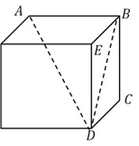To find the edge, calculate the right triangles until you reach dotted line AD.

1. Call the edge e , which represents the lengths of edges BC, CD, DE, BE, and AB in the drawing.

2. With BCD as a right triangle, find the length of dotted line BD: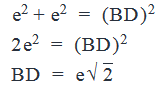3. Now you have a new right triangle ABD, where AB = e, BD = e√2, and hypotenuse AD = √15 (from the statement). Set up the equation to solve for e: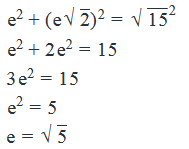And from this, you can calculate the cube volume of 5√5

Statement (2) is sufficient.

Step 5: Analyze Both Statements Together (if needed)

You get unique answers in steps 3 and 4, so this step is not required

Test: Rectangular Solids- 1 - Question 9

Two cylindrical buckets – A and B – contain only water. Three-fourths of bucket A’s volume is occupied by water while one-fourths of bucket B’s volume is occupied by water, When all the water in bucket A is poured into bucket B, bucket B gets exactly half-filled. If the radius of the base area of bucket A is twice the radius of base area of bucket B, and the height of bucket B is 2 metres, what is the height of the container A in metres?

Detailed Solution for Test: Rectangular Solids- 1 - Question 9

Step 1: Question statement and Inferences

Let the radius, height and volume of bucket A be r, h and v. Let the radius, height and volume of bucket B be R, H and V.

Since three-fourths of bucket A raises the volume of bucket B rise from one-fourths to one-half of its volume,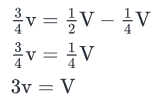ividing both sides of the equation by , we get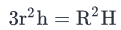Step 2: Finding required values

We also know that r = 2R and H = 2. Substituting these values and, we have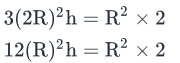Step 3: Calculating the final answer

Dividing both sides by R2, we obtain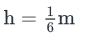Test: Rectangular Solids- 1 - Question 10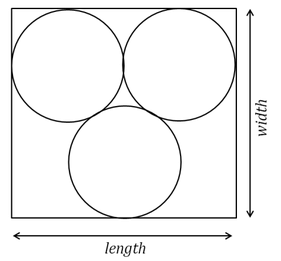Three identical cylinders are inscribed within a rectangular solid, shown above from the top. If the length of the rectangular solid is 4 feet and the height (not shown) is 2 feet, what is the volume of the rectangular solid?

Detailed Solution for Test: Rectangular Solids- 1 - Question 10

Step 1: Question statement and Inferences

For the volume of the rectangular solid, you need the length, width, and height. You already have the length and the height, 4 and 2, respectively. Use the cylinders to calculate the width.

Step 2: Finding required values

Draw an equilateral triangle where each vertex is the center of one circle.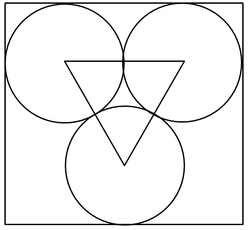If the length of the rectangle is 4, the diameter of each circle is 2, and the radius is 1. The triangle is equilateral with a base of 2.

To find the height of the triangle, cut it in half as shown below, into two 30-60-90 triangles. With a hypotenuse of 2 and a base of 1 (half the side is the base), use the Pythagorean Theorem to find the third side, which is the height: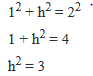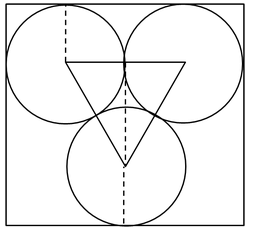Add this height of the triangle to the circle radii above and below it for a rectangular solid width of (2 + √3)

Step 3: Calculating the final answer

By multiplying the length and height by the newly found width, we get:

The volume of the solid =  4*2*(2 + √3)

= 8*(2 + √3)

Test: Rectangular Solids- 1 - Question 11

If right circular cylinders A and B have the same radius : height ratio,  what is the ratio of the curved surface area of cylinder A to the curved surface area of cylinder B?

(1)          The ratio of the radius of cylinder A to the radius of cylinder B is 2:1

(2)          The ratio of the volume of cylinder A to the volume of cylinder B is 8:1

Detailed Solution for Test: Rectangular Solids- 1 - Question 11

Steps 1 & 2: Understand Question and Draw Inferences

Let the radius and height of Cylinder A be RA and HA respectively, and

The radius and height of Cylinder B be RB and HB respectively.

Given:

RA: HA = RB: HB

This equation can also be written as:

RA: RB = HA : HB  . . . (1)

We know that the curved surface area of a right circular cylinder, CSA = 2πRH, where R and H are the radius and height of the cylinder respectively.

So, CSAA : CSAB = 2πRA HA : 2πRB HB

CSAA : CSAB = RA HA : RB HB   . . . (2)

Substituting (1) in (2), we get:

CSAA : CSAB = RA 2: RB2 = HA 2: HB2  . . . (3)

From Equation (3) it is clear that we will be able to determine the ratio of CSAA : CSAB if we know the ratio RA  : RB  or the ratio HA  : HB

Step 3: Analyze Statement 1

(1)         The ratio of the radius of cylinder A to the radius of cylinder B is 2:1

Since the ratio RA  : RB  is given, we will be able to determine the ratio CSAA : CSAB

Statement 1 is sufficient.

Step 4: Analyze Statement 2

(2)         The ratio of the volume of cylinder A to the volume of cylinder B is 8:1

We know that volume of a right circular cylinder, V = πR2 H, where R and H are the radius and height of the cylinder respectively.

So,

VA : VB = πRA 2HA : πRB 2HB

VA : VB = RA 2HA : RB 2HB  . . . (4)

Substituting (1) in (4), we get:

VA : VB = RA 3: RB3   . . . (5)

We are given that VA : VB = 8:1

So, from Equation (5), we get:

RA 3: RB3   = 8:1

This means that,

RA  : RB  = 2:1

Since we have been able to determine the ratio RA  : RB  is given, we will be able to determine the ratio CSAA : CSAB

Statement 2 is sufficient.

Step 5: Analyze Both Statements Together (if needed)

You get a unique answers in steps 3 and 4, so this step is not required

Test: Rectangular Solids- 1 - Question 12

If the length of each edge of a certain rectangular solid is an integer and exactly four of its faces have the same dimensions, then what is the volume of the rectangular solid?

(1) Two of the faces have areas of 32 square units and 16 square units.

(2)  One of the edges is twice the length of another edge.

Detailed Solution for Test: Rectangular Solids- 1 - Question 12

Steps 1 & 2: Understand Question and Draw Inferences

Some general points to be noted:

(a) In a rectangular solid, each pair of adjacent faces has one common edge.

(b) The opposite faces have the same dimensions.

Some inference that can be drawn from the question:

(c) Since ‘exactly’ four faces have the same dimensions, the other two faces must have different dimensions.

(d) Two of the faces which have different dimensions compared the four faces which have the same dimensions must have the following properties:

(i) They must be opposite to each other and their areas must be equal.

(ii) They must both be of the shape of a square.

(iii) Each of these two faces has a common edge with each one of the other four faces.

(e) The lengths of edges are positive integers.

Step 3: Analyze Statement 1

The possible values of the lengths and breadths for the face whose area is 32 sq. units are: 32 and 1, OR 16 and 2 OR 8 and 4.

The possible values of the lengths and breadths for the face whose area is 16 sq. units are: 16 and 1 OR 8 and 2 OR 4 and 4.

Since one of the surfaces must be a square and the lengths of edges are integers, between the two areas given only the face with area of 16 sq. units makes a square. There will be only two such surfaces in this solid with edge lengths 4 units each.

The only possible lengths of the faces whose areas are 32 square units are 8 units and 4 units.

We now know all the dimensions of the rectangular solid (8 units, 4 units, 4 units) and hence its volume can be found out.

SUFFICIENT.

Step 4: Analyze Statement 2

Let’s say that the square face has edges of lengths ‘a’ units each. This means that the length of the other side of the rectangular faces is ‘2a’ or ‘a/2’. (Depending on which length is the bigger one.)

Since we do not have the values of the edges or any other numeric information, we cannot ascertain the volume of this rectangular solid.

INSUFFICIENT.

Step 5: Analyze Both Statements Together (if needed)

Since the answer has been obtained in Step 3, there is no need to combine the statements.

Test: Rectangular Solids- 1 - Question 13

What is the greatest possible (straight-line) distance between any two points on a certain rectangular solid of length L, width W, and height H, where H < W < L ?

(1) The length, width, and height of the rectangular solid are the squares of prime integers.

(2) The roots of the equation x2 – 13x = -36 are the width and the height of the rectangular solid.

Detailed Solution for Test: Rectangular Solids- 1 - Question 13

Steps 1 & 2: Understand Question and Draw Inferences

For the longest straight-line distance between any two points, you need the length, width, and height of the rectangular solid.  You know that the height, the width, and the length are in ascending order of magnitude.

Step 3: Analyze Statement 1

(1) The length, width, and height of the rectangular solid are the squares of prime integers.

This narrows the possibilities: L, W, and H could be 4, 9, 25, 49, and so forth, but there are still too many possibilities.

Statement (1) is not sufficient.

Step 4: Analyze Statement 2

(2) The roots of the equation x2 – 13x = -36 are the width and the height of the rectangular solid.

Factor the expression to (x – 9)(x – 4) for the roots. Thus the height and the width are 4 and 9, respectively (Since H < W).

However, knowing H < W < L, the length could be any number greater than 9.

Statement (2) is not sufficient.

Step 5: Analyze Both Statements Together (if needed)

You know from the question that H < W < L.

You know from Statement 1 that L, W, and H could be 4, 9, 25, 49, and so forth.

You know from Statement 2 that H and W are 4 and 9, respectively.

Taken together, L could be 25, 49, 121, or the square of any prime number greater than 3.

Hence both the statements together are not sufficient.

Test: Rectangular Solids- 1 - Question 14

A 4 cm cube is cut into 1 cm cubes. What is the percentage increase in the surface area after cutting?

Detailed Solution for Test: Rectangular Solids- 1 - Question 14

Volume of 4 cm cube = 64 cc. When it is cut into 1 cm cube, the volume of each of the cubes = 1cc
Hence, there will be 64 such cubes. Surface area of small cubes = 6 (12) = 6 sqcm.
Therefore, the surface area of 64 such cubes = 64 * 6 = 384 sqcm.
The surface area of the large cube = 6(42) = 6*16 = 96.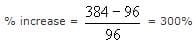Test: Rectangular Solids- 1 - Question 15

A regular hexagon is inscribed in a circle of radius r cm. What is the perimeter of the regular hexagon?

Detailed Solution for Test: Rectangular Solids- 1 - Question 15

A regular hexagon comprises 6 equilateral triangles, each of them having one of their vertices at the center of the hexagon. The sides of the equilateral triangle are equal to the radius of the smallest circle inscribing the hexagon. Hence, each of the side of the hexagon is equal to the radius of the hexagon and the perimeter of the hexagon is 6r.

## Quantitative for GMAT

88 videos|99 docs|165 tests
 Use Code STAYHOME200 and get INR 200 additional OFF Use Coupon Code
Information about Test: Rectangular Solids- 1 Page
In this test you can find the Exam questions for Test: Rectangular Solids- 1 solved & explained in the simplest way possible. Besides giving Questions and answers for Test: Rectangular Solids- 1, EduRev gives you an ample number of Online tests for practice

## Quantitative for GMAT

88 videos|99 docs|165 tests